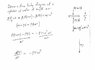# Finding pressure with given radius

• Acuben

## Homework Statement

http://img585.imageshack.us/img585/613/labnotebookq.gif [Broken]

A Test tube filled with water i being spun around in an ultracentrifuge with constant angular velocity, w (w = omega). The test tube i slying along a radius and revolving in a horizontal plane. The free surface of the water is at radius r(0) (with smaller parenthesis) as shown

a) show that the pressure at any raidus r within the tube is given by: P=1/2 (row)(omega)^2+P(atm)

b)suppose that w=3.8x10^5 rad/s and r(0)=10cm, what is pressure at r=13
Does it matter whether it's gauge or atmospheric pressure?

question, is it asking whether gauge pressure and actually pressure are the same?

## Homework Equations

centripetal accleration =v^2/r = r(omega)^2
Pressure= P(atm) + (row)*g*h
where row is density, g is gravity (or acceleration in this case), and h is dept
density = (row) = m/V = mass over volume

## The Attempt at a Solution

took simple approach
a= r(omega)^2

and used this "a" (acceleration) as"g" (gravity)
and r-r(0) (since that's the dept of watr) as h
so using Pressure= P(atm) + (row)*g*h
P= P(atm) + (density of water)*r*(omega)^2*(r-r(0))
in symbols :P
P= P(atm)+p*r*w^2(r-r(0))
but this doesn't match the answer ;)

Last edited by a moderator:

•